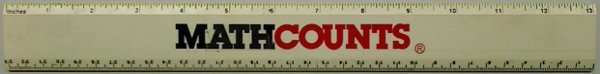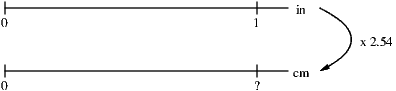### Modeling Dimensional Analysis

I generally ask myself two questions when I examine the design of a mathematical task:
1. What is the context?
2. How can we model the mathematics?
Mathematical concepts with tasks for which these two questions can be answered easily tend to be easier to learn, while teaching and learning generally becomes more difficult when one or both of those questions can't be answered. For dimensional analysis (sometimes called the unit factor method or the factor-label method), the first question is easy to answer. It doesn't take much of an imagination to design a measurement conversion task that is set in a real-world context. A model, however -- whether visual, mental, or a concrete manipulative -- is generally absent. Typical dimensional analysis problems look like this:

Q: What is 60 miles per hour in meters per second?

A: $$\frac{60 \mbox{mi}}{1 \mbox{hr}} \times \frac{5280 \mbox{ft}}{1 \mbox{mi}} \times \frac{12 \mbox{in}}{1 \mbox{ft}} \times \frac{2.54 \mbox{cm}}{1 \mbox{in}} \times \frac{1 \mbox{m}}{100 \mbox{cm}} \times \frac{1 \mbox{hr}}{60 \mbox{min}} \times \frac{1 \mbox{min}}{60 \mbox{sec}} = \frac{9656064 \mbox{m}}{360000 \mbox{sec}} = \frac{26.8224 \mbox{m}}{\mbox{sec}}$$

For those who successfully learn dimensional analysis this way, there's a certain beauty to how the units drive the problem and how the conversion factors are nothing more than cleverly written values of one, the multiplicative identity. Unfortunately, many students struggle with this method. Some are intimidated by the fractions, some can't get the labels in the right place, and some just can't get the problem started.

What we need is a model. Let's start with the most basic of unit conversion models, a ruler with both inches and centimeters:(Yes, I'm still using the same ruler I got as a 7th grader in a regional MathCounts competition.)
With only simple visual inspection, students should be able to use a ruler to estimate conversions between inches and centimeters. This is an informal model, one students can literally get their hands on. We can assist the learning by making the models progressively more formal. Here we model a trivial conversion from one inch to centimeters with a double number line:(Yes, you still have to know your conversion factors!)
Such a simple example looks almost too easy to be useful, but we can add number lines for more complex conversions. We can even abstract the model further and go beyond conversions of distance. Suppose we wanted to convert 3 gallons to liters. I could model that conversion with number lines this way:(I could have used any number of transition units, but I knew 1 quart was roughly 946 milliliters.)
Filling in the question marks from top to bottom, I'll see that 3 gallons, 12 quarts, 11,352 milliliters, and 11.352 liters are all the same volume. It's easy to see they're the same because on each number line those values are the same distance from zero. Because we're only converting one kind of unit (volume), we only need one dimension.

In our initial example we were converting 60 miles per hour to meters per second. That's two kinds of units, distance and time, so our model needs two dimensions. Furthermore, it can help to think of 60 miles per hour as a line, not just a point. After all, we often travel at a speed of 60 miles per hour without actually traveling a distance of 60 miles in exactly one hour.
Can you guess where our double (or however many are necessary) number lines will go in this model? The following video will demonstrate what I would call the graphing model or two dimensional model for performing conversions.
With the work shown in the video, we haven't just done one conversion. In fact, we're prepared to write 60 miles per hour 15 different ways, not that we'll ever be asked to do that. If we needed 60 miles per hour in centimeters per minute or feet per second, all the work is done. Just choose the appropriate quantity from the vertical and divide by the appropriate quantity from the horizontal. Of course, if we're in a hurry, we won't find all those intermediate figures and instead just proceed from miles to meters and hours to seconds as quickly as possible. Will that be quicker than the traditional method shown above? Probably not, but the purpose of using a model is understanding, not speed. Once the understanding is established, students can move on to a formal method or use technology when appropriate.